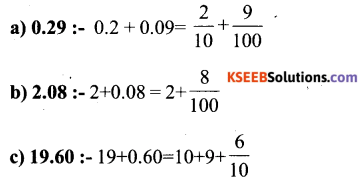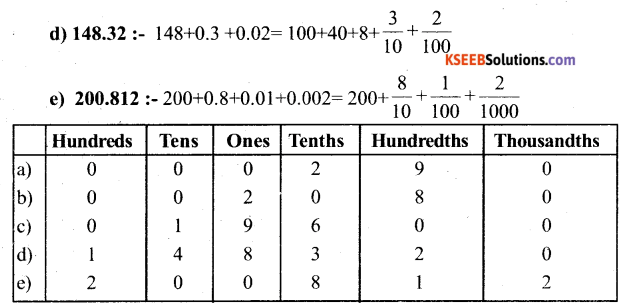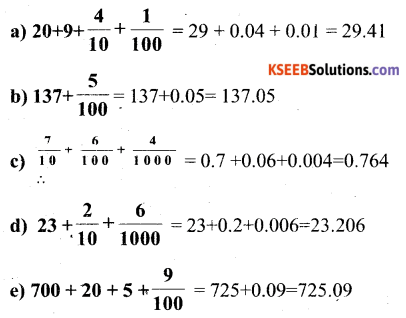# KSEEB Solutions for Class 6 Maths Chapter 8 Decimals Ex 8.2

Students can Download Chapter 8 Decimals Ex 8.2 Questions and Answers, Notes Pdf, KSEEB Solutions for Class 6 Maths helps you to revise the complete Karnataka State Board Syllabus and score more marks in your examinations.

## Karnataka State Syllabus Class 6 Maths Chapter 8 Decimals Ex 8.2

Question 1.
Complete the table with the help of these boxes and use decimals to write the number.Solution:Question 2.
Write the numbers given in the following place value table in decimal formSolution:Question 3.
Write the following decimals in the place value table.
a) 0.29
b) 2.08
c) 19.60
d) 148.32
e) 200.812.
Solution:Question 4.
Write each of the following as decimals.Solution:Question 5.
Write each of the following decimals in words.
a) 0.03
b) 1.20
c) 108.56
d) 10.07
e) 0.032
f) 5.008
Solution:
a) 0.03 = Zero point zero three
b) 1.20 = One point two zero
c) 108.56 = One hundred eight point five six
d) 10.07 = Ten point zero seven
e) 0.032 = Zero point zero three two
f) 5.008 = Five point zero zero eightQuestion 6.
Between Which two numbers in tenths place on the number line does each of the given number lie?
a) 0.06
b) 0.45
c) 0.19
d) 0.66
e) 0.92
f) 0.57
Solution:
a) 0.06 = 0 and 0.6
b)0.45 = 0.4 and 0.5
c) 0.19 = 0.1 and 0.2
d) 0.66 = 0.6 and 0.7
e) 0.92 = 0.9 and 1.0
f) 0.57 = 0.5 and 0.6Question 7.
Write as fractions in lowest terms.
a) 0.06
b) 0.05
c) 0.75
d) 0.18
e) 0.25
f) 0.125
g) 0.066
Solution:error: Content is protected !!